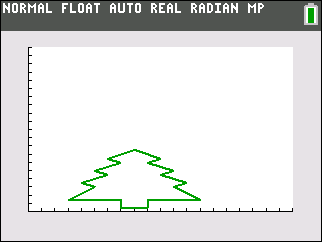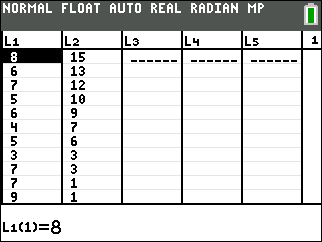••• ##### Device
• TI-83 Plus Family
• TI-84 Plus
• TI-84 Plus Silver Edition
•TI-84 Plus C Silver Edition
•TI-84 Plus CE

TI Connect™

# Middle Grades Math: Changing Before My Eyes

by Texas Instruments#### Overview

In this activity, students will discover how pictures formed by graphing ordered pairs can be stretched (and shrunk) by multiplying (and dividing) the coordinates.

#### Key Steps

•Students will discover how pictures formed by graphing ordered pairs can be stretched (and shrunk) by multiplying (and dividing) the coordinates.

First, students will identify the coordinates of each point of the given image, enter the x- and y-values into L1 and L2, and graph.

•Then, students will double the x-values in each ordered pair and observe the change in the graph. Next, they will manipulate the y-values and observe the changes. Finally, students will make predictions about the impact different multipliers will have on the image.